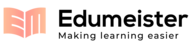## Lec37.pdf-Fermions and Bosons Symmetry is ashowing page 1-5 out of 5

##### Page 1
Fermions and Bosons
Symmetry is a constant theme in physics. As a fundamental
symmetry result of QM, particles divide into two classes
according to their
spin
:
http://
en.wikipedia.org
/wiki/
Identical_particles
fermions
:
half-integer spin
e.g., electrons, protons, neutrons,
3
He
bosons
:
integer spin
0,1,2,3...
e.g., photons,
4
He
The two classes have profoundly different quantum rules for
the states they may be in, for non-interacting particles
1)
any integral number of bosons of the same species
(0,1,2...) may be described by the same quantum state
2)
for fermions of the same species, at most one particle,
i.e., (0, 1), may be described by a given state
1
2
,
3
2
,
5
2
...
http://
en.wikipedia.org
/wiki/
Identical_particles#Symmetrical_and_antisymmetrical_states

##### Page 2
Fermi-Dirac Distribution
Consider a ‘system’ of one orbital or wavefunction
Ψ
It may contain zero fermions, or one fermion.
Take the
energy as 0 for zero fermions, and
ε
for one fermion.
then
so we can find the thermal average state occupation for a
system in diffusive and thermal contact with the reservoir:
or conventionally, for the average occupancy:
the
Fermi-Dirac distribution function
Z
exp (
N
μ
ε
s
(
N
)
)/
τ
s
N
=
0
1
λ
exp(
μ
/
τ
)
'activity'
N
(
ε
)
=
N
(
ε
)
P
(
ε
)
=
λ
exp(
ε
/
τ
)
1
+
λ
exp(
ε
/
τ
)
=
1
λ
1
exp(
ε
/
τ
)
+
1
f
(
ε
)
N
(
ε
)
1
exp(
ε
μ
(
)
/
τ
)
+
1
=
1
+
exp (
μ
ε
)/
τ
[
]
=
1
+
λ
exp(
ε
/
τ
)

##### Page 3
Bose-Einstein Distribution
Now, not just 1 but some arbitrary number
N
of bosons can
have the same wavefunction
Ψ
(or we say we ‘populate’ the
orbital with
N
photons). The energy of each added boson is
ε
. In diffusive and thermal equilibrium with a reservoir
thus
or conventionally, for the average occupancy:
the
Bose-Einstein distribution function
using
x
N
N
=
0
=
1
1
x
for
x
<
1, always true here
Z
exp (
N
μ
N
ε
)/
τ
[
]
N
=
0
=
exp (
μ
ε
)/
τ
[
]
N
N
=
0
=
1
1
exp
μ
ε
(
)
/
τ
{
}
N
(
ε
)
N
(
ε
)
P
(
ε
)
=
N
exp (
N
(
μ
ε
)/
τ
[
]
N
=
0
Z
=
1
exp(
ε
μ
(
)
/
τ
)
1
f
(
ε
)
N
(
ε
)
=
1
exp(
ε
μ
(
)
/
τ
)
1

##### Page 4
Fermi-Dirac Distribution
http://commons.wikimedia.org/wiki/User:Krishnavedala